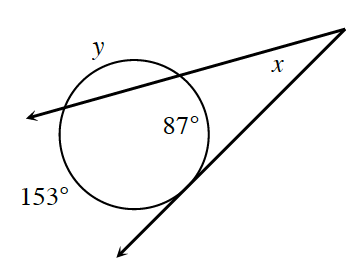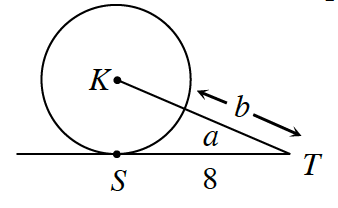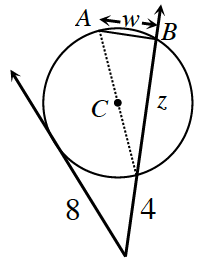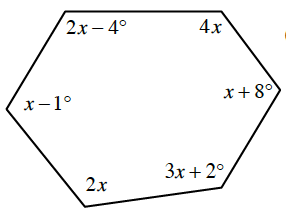### Home > CCG > Chapter 12 > Lesson 12.1.4 > Problem12-52

12-52.

Use the relationships in each diagram below to solve for the given variables.

1.The secant-tangent angle is half the difference between the major and minor arcs.

$x\ =\ \frac{153 - 87}{2}$

The total measure of all the arcs in a circle is 360°.

$153^\circ + 87^\circ + y = 360^\circ$

$y\ =\ 120^\circ \ \ \ x\ =\ 33^\circ$

1. The area of $\odot K$ is $36\pi$ sq. units.The area of a circle is $r^2\pi$.

$\overline{KS}$ is the radius of $\odot K$.

$a \approx 36.9^\circ, \ b\ =\ 4$

1. The diameter of $\odot C$ is $13$ units. $w$ is the length of $\overline{AB}$.1.Refer to the Math Notes box in Lesson 8.1.4 for help calculating the sum of all of the angles in a polygon.